• 本书为模型参考自适应控制教材，可供有需求者下载学习。
•研究论文
• 针对典型的鲁棒模型参考自适应控制中瞬态性能无法得到保障的问题, 提出一种改进的鲁棒模型参考自适应控制器. 该控制器在标准的鲁棒自适应控制中加入??∞ 补偿器, 以抑制闭环自适应系统中参数估计误差和不确定扰动对...
• 模型参考自适应控制系统的设计主要有两大类方法：一种是基于局部参数最优化的设计方法；另一种是基于稳定性理论的设计方法，包括以下两种具体的设计方法： 基于Lyapunov稳定性理论的方法； 基于Popov超稳定性理论...
一、模型参考自适应

模型参考自适应是比较流行的自适应控制方式之一。模型参考自适应控制系统的设计主要有两大类方法：一种是基于局部参数最优化的设计方法；另一种是基于稳定性理论的设计方法，包括以下两种具体的设计方法：

基于Lyapunov稳定性理论的方法；
基于Popov超稳定性理论和正实性概念的方法。
早期的自适应控制大多采用局部参数最优化的设计方法，其主要缺点是在整个自适应过程中难以保证闭环系统的全局稳定性。而基于稳定性理论的设计方法，则从保证系统稳定性的角度出发来选择自适应规律， 因此易于保证系统的稳定性。

1.1  模型参考自适应控制系统的典型结构

系统由参考模型、可调系统、自适应机构三部分构成。

可调系统包括被控对象、前置控制器和反馈控制器。
对可调系统的特性要求，如超调量、阻尼性能、过渡时间和通频带等由参考模型规定，故参考模型实际上是一种理想控制系统，其输出代表了期望的性能。
当参考模型与实际被控对象的输出有差异时，经比较器检测后，通过自适应机构做出决策，改变调节器（包括前置和反馈控制器）参数或生成辅助输入，以消除误差，使过程输出和参考模型输出一致。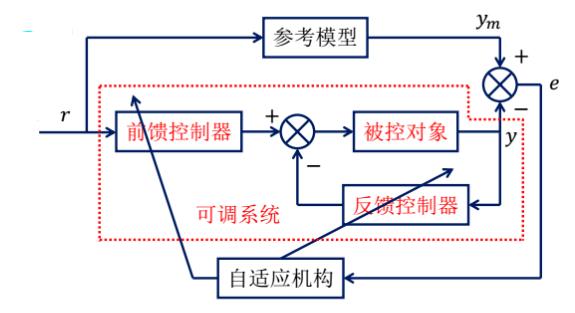参考模型与可调系统两者性能之间的一致性，由自适应机构保证，所以自适应机构的设计十分关键，性能一致性程度由状态广义误差向量

或输出误差向量

度量，其中和分别为参考模型和可调系统的状态和输出。

只要误差向量不为零，自适应机构就按减少误差方向修正或更新控制律，以使系统实际性能指标达到或接近希望的性能指标。具体实施时，可更新前置和反馈控制器的参数，也可直接改变加到对象输入端的信号。前者称为参数自适应方案，后者称为信号综合自适应方案。

1.2  模型参考自适应控制系统的结构类型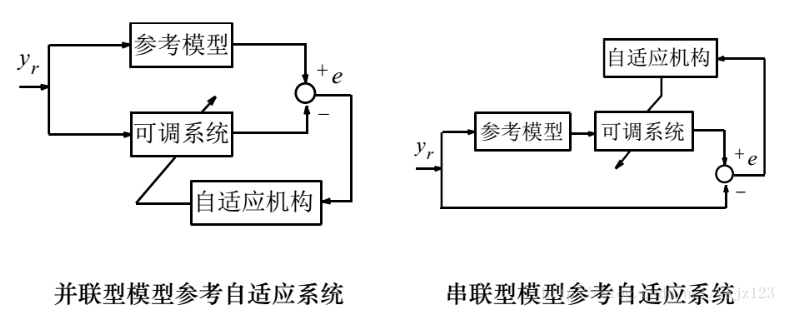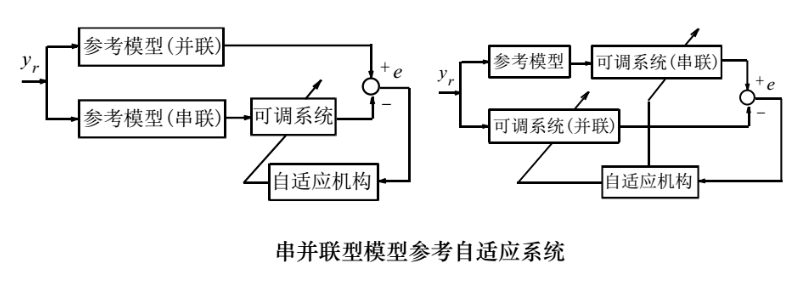注：在这三种结构中，并联型方案是最普遍的结构。尽管这三种结构的形式不同，但实际上对它们进行分析和综合的方法基本相同，故本节将限于讨论并联型模型参考自适应系统。

关于模型参考自适应控制系统的假定

参考模型是时不变系统；
参考模型和可调模型是线性的，有时为了分析方便，还假设它们的阶次相同；
广义误差可测；
在自适应控制过程中，可调参数或辅助信号仅依赖于自适应机构。
注：由于环境干扰引起的系统参数的变化相对于自适应调节速度要慢得多，故自适应速度快于对象参数的变化速度。假设4意味着自适应速度应大于被控对象参数的变化速度，否则就不可能实现渐进自适应。

1.3  基于局部参数最优化的设计方法（MIT方案）

局部参数最优化方法的设计思想：系统包含若干可调参数（如可调增益、反馈回路可调参数等），当被控对象的特性由于外界环境条件的改变或其他干扰的影响而发生变化时，自适应机构对这些可调参数进行调整，以补偿外界环境或其他干扰对系统性能的影响，从而逐步使得参考模型和控制对象之间的广义误差所构成的性能指标达到或接近最小值，因此它的设计原理就是构造一个由广义误差和可调参数组成的目标函数，并把它视为可调参数空间中的一个超曲面，利用参数最优化方法使这个目标函数逐渐减小，直到木目标函数达到最小或位于最小值的某个领域为止，从而满足可调系统与参考模型之间的一致性要求。

额外两条假设

可调系统参数已位于参考模型参数的某个邻域内；
相对于系统的内部动力学时间尺度而言，可调参数的调节速度低，即自适应增益小。
考虑具有一个可调增益的模型参考自适应控制系统，假设被控对象的传递函数为：

其中，和为已知的常系数多项式，为对象的增益。

问题的背景

当系统受到干扰时，被控对象的增益可能发生变化，使其动态特性与参考模型的动态特性之间发生偏离，的变化是不可测量的。为了克服由的漂移所造成的影响，在控制系统中设置一个可调增益，来补偿由的变化所造成的影响，期望使得与的乘积始终与模型的增益相一致。那么，如何设计自适应机构来实时地调整，即如何设计的自适应调整规律是需要解决的问题。

解决方法

构造理想参考模型的传递函数为：

其中，增益是常数，认为是已知的，通常由期望的动态响应决定。

控制系统的结构图：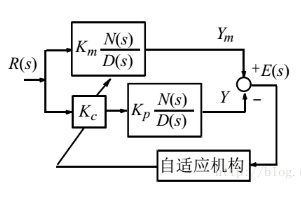定义广义输出误差为：

其中，为理想参考模型的输出；为被控系统的输出；表示输入信号为时，理想系统的响应与实际系统响应之间的偏离。

设计目标：确定可调增益的自适应调节律，使得下列性能指标达到最小

（1.1）

采用梯度法来寻求的最优调节律。

首先求对的偏导数有：

根据梯度下降原理，的变化量应正比于函数的负梯度方向，即取如下数值：

其中，，则调整后的为：

其中，为可调增益的初值。

将上式两边分别对时间求导数后，得到的变化率与广义误差的关系为：
（1.2）

上式表示了可调增益的自适应调整规律，只要求出，增益调整律就可确定。为此，需要确定关于的函数关系。

由系统结构图可知，参考输入到输出偏差的传递函数为：

将上述拉普拉斯变换转化为微分方程描述的时域算子形式，即令：

则可得到所满足的微分方程为：

上式两端对求导数为：
（1.3）

另一方面，考虑到参考模型的输出与输入之间满足下列关系：
（1.4）

令（1.3）式与（1.4）相除，并整理得：

将此式代入（1.2）式有：

令，则有：

（1.5）

（1.5）式就是所求的可调增益的调节律，也就是系统的自适应规律。这种自适应规律最先由麻省理工学院提出，故又称为MIT自适应规律。

由（1.5）式可以看出，为实现这种自适应律，自适应机构由一个乘法器和一个积分器组成，具体实现的结构图如下：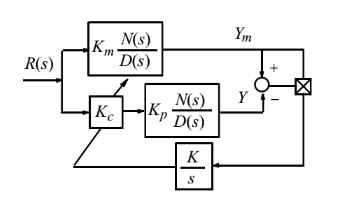这样综合出来的模型参考闭环自适应系统的数学模型可用下列一组方程来描述：

其中， 。

MIT方案的特点：

该方案利用的是输出偏差，而不是状态偏差，所以自适应律所需的信号都是容易获得的，这是MIT方案额的主要优点。
这种设计方法在设计过程中并未考虑稳定性问题，不能保证所设计的自适应控制系统总是稳定的， 这是它的缺点。因此，在求得自适应规律后，尚需进行稳定性校验，以确保广义误差在闭环回路中能收敛于某一允许的数值。
【举例1】

考虑一个一阶系统，其传递函数为。根据MIT规则设计的闭环自适应控制系统的数学模型为：

假定在时，和均为零，且。试考察该自适应控制系统的稳定性。

【解】

假定在时给定系统输入一个幅度为的阶跃信号，则之后参考模型的输出为：

所以自适应调节律为：

对开环广义误差方程求导数得：

或

当时，上式右端第三项的系数趋于，即有：

此系统方程是渐近稳定的，即时，有：和。（根据劳斯判据）

结论：
对于一阶系统，按照MIT规则设计的闭环自适应系统总是稳定的。

跟踪速度或自适应速度是按指数规律进行的。理论上说，仅当时，误差才趋于零，所以自适应速度是比较慢的。实际应用中，并不要求完全等于0。当（为一很小的选定值）时，就认为系统已跟上参考模型了。在此意义上，自适应调整时间还是有限的。

【举例2】

设一个被控对象的传递函数为，理想参考模型为。根据MIT规则设计的闭环自适应控制系统的数学模型为：

假定在时给系统输入一个幅度为的阶跃信号，即。我们来研究偏差的稳定性。

对上式偏差微分方程的两端求导，并整理得：

假设的动态响应比的自适应调整过程快得多。也就是说，在研究的调节过程时，认为已达到了它的稳定值，那么的微分方程就可简化为：

利用劳斯（Routh）稳定性判断，易知当

时，系统不稳定。也就是说，当满足条件上式时，输出偏差将出现不稳定。

上例说明，在应用局部参数最优化方法进行设计时，需要对整个系统的稳定性进行分析和检验，然而这一步工作往往是很麻烦的。

时的劳斯判据：时，特征方程为：，各系数为正。时，特征方程为：，各系数为正。

时，特征方程为：，各系数为正，且。

时，特征方程为：，各系数为正，且。

展开全文• 考虑带有未知回滞驱动且以状态空间形式表示的连续时间线性动态系统, 给出了模型参考自适应控制设计方案.控制策略保证闭环系统的全局稳定性和期望的跟踪精度,有效地抑制回滞产生的不精确和振荡现象.数值仿真结果表明...
• 的神经网络模型参考自适应控制器设计方案, 简化了基于神经网络的模型参考自适应控制系统的设计。 给出了统一的设计步骤, 它适用于任意非线性系统, 更接近于工程实际。理论分析和仿真结果证明了该 方案的...
• 离散时间直接模型参考自适应控制在高精度惯性稳定平台中的应用研究论文
• 针对任意复杂的具有最小相位，滞后环节和非最小相位特性的离散非线性系统，提出一种通用的直接神经网络模型参考自适应控制。采用具有在线学习功能的最近邻聚类算法训练RBF神经网络控制器，同时引入优化策略对聚类...RBF神经网络 最近邻聚类算法
• 提出一种模型参考自适应控制系统. 该系统只要求知道对象阶次的上界, 不要求对象严格正实和最小相位, 并且允许存在建模误差和作用在对象任何部位的大幅值高频干扰. 系统的强鲁棒性来源于特殊的系统结构和对信号...
• 基于RBF网络辨识的模型参考自适应控制系统分析与仿真论文资料
• 现主动控制，采用基于李雅普诺夫稳定性理论设计模型参考自适应控制；仿真结果表明，基于李雅普诺夫稳 定性理论设计的模型参考 自适应控制效果比直接速度反馈好，稳定性和动态性能均有较大提高。
• 内容包括：实例，文档分析，设计过程和MATLAB仿真程序。首先利用BP网络对以下非线性系统进行辨识，然后利用模型参考自适应方法，设计NNMARC控制
• 针对机械臂运动轨迹控制中存在的跟踪精度不高的问题，采用了一种基于EC-RBF神经网络的模型参考自适应控制方案对机械臂进行模型辨识与轨迹跟踪控制。该方案采用了两个RBF神经网络，运用EC-RBF学习算法，采用离线与...
• 3 = 3 具有噪声的一类简单系统, 给出了具有未规范化自适应律的鲁棒直接型模型参考自适应 控制器的设计. 通过引入非线性阻尼项, 保证了闭环系统的所有信号都是全局稳定的, 而且跟踪误差及参数估计误差 均收敛...文档
• 鲁棒模型参考自适应控制_1 引言 在设计一个控制系统时,其闭环稳定性,指令跟踪能力以及应对各种不确定因素(如模型误差,环境扰动,信号噪声等)的鲁棒性都是非常重要的性能参考指标.然而,当我们的基线控制器运行在充满不...
模型参考自适应控制(MRAC)
引言
在设计一个控制系统时,其闭环稳定性,指令跟踪能力以及应对各种不确定因素(如模型误差,环境扰动,信号噪声等)的鲁棒性都是非常重要的性能参考指标.然而,当我们的基线控制器运行在充满不确定性的环境中时,其各项性能指标都不可避免地遭受不同程度的损失. 因此,我们提出这样一个问题:
是否可以通过某种控制器设计方法,提升控制器在充满不确定因素的环境中运行的性能,甚至恢复至与基线控制器在理想条件下运行的性能相当的水平?
基于这个问题,我们引入模型参考控制的概念.其设计理念是通过设计一个稳定的,理想条件下可预测的,满足我们需求的系统模型作为参考模型,再利用实际信号和参考模型信号之间的残差驱动着去修正最终控制律,使得实际的闭环系统特性趋近于预设的理想模型特性,即达到模型跟踪的效果.在这个过程中,理想模型中是不存在不确定因素的,因此模型参考控制也可以达到减少实际系统中不确定因素带来的性能损失的作用.
问题方程建立
不失一般性的,我们将存在不确定度的开环系统以以下形式建立状态空间模型
$\dot{x}_p=A_px_p+B_p\Lambda u +B_p\delta_p(x_p)$
其中 $A_p,B_p$ 分别是系统矩阵与控制矩阵, $x_p$ 是系统状态量,$u$ 是系统控制律.

上述状态空间模型的维度信息如下:
\begin{aligned} x_p &\in \Re^{n_p \times 1} \\ A_p &\in \Re^{n_p \times n_p} \\ B_p &\in \Re^{n_p \times m} \\ \Lambda &\in \Re^{m \times m} \\ u &\in \Re^{m \times1} \\ \delta_p &\in \Re^{m \times 1} \\ \end{aligned}
$\Lambda$ 被定义为已知的控制有效性不确定度,其形式如下
$\Lambda = \left[ \begin{matrix} \Lambda_1 & 0 & \cdots & 0 \\ 0 & \Lambda_2 & \cdots & 0 \\ \vdots & \vdots & \ddots & \vdots \\ 0 & 0 & \cdots & \Lambda_m \\ \end{matrix} \right] \succ0$
$\delta_p(x_p)$ 被定义为系统非线性不确定度,其形式如下
\begin{aligned} &\delta_p(x_p)=W_p^T \sigma_p(x_p)\\& \end{aligned}
其维度信息如下:
\begin{aligned} \delta_p &\in \Re^{m \times 1}\\ W_p &\in \Re^{n_s \times m}\\ \sigma_p &\in \Re^{n_s \times 1} \end{aligned}
其中 $W_p$ 是未知的权重,$\sigma_p$ 是已知的基函数,其形式如下
\begin{aligned} &\sigma_p(x_p)=[\sigma_{p_1}(x_p) \ \cdots \ \sigma_{p_s}(x_p)] \\ &\sigma_{p_i}: \Re^{n_p\times1} \to \Re^{n_s\times1},\text{ a locally lipschitz function} \end{aligned}
因此,我们可以将系统的状态空间方程重写为
$\dot{x}_p=A_px_p+Bp\Lambda[u + \Lambda^{-1}B_p\delta_p(x_p)]$
值得注意的是,上述方程的形式告诉我们,控制律 $u$ 可以访问所有的不确定度. 那么,这是否意味着我们可以通过设计控制律 $u$
来达到消除系统不确定度带来的影响的目的呢? 先不着急,带着这个问题,我们先在不考虑不确定度的前提下为这个开环系统设计一个基线控制器.
首先,我们将整体控制律 $u$ 定义为以下的形式
$u = u_n + u_a$
其中 $u_n$ 是在理想(即模型准确,不存在不确定因素)的条件下为开环系统设计的控制律,称为基线控制律.
$u_a$ 则是为消除系统不确定度影响而设计的控制律,称为自适应控制律.
现在,我们就为这个理想系统设计基线控制器,在这种情况下 $u_a = 0$,我们将基线控制律 $u_n$设计为以下形式
$u = u_n + u_a = u_n = -K_xx_p+K_rr$
其中 $K_x$ 为反馈增益,&x_p&为反馈的状态量,$K_r$ 为前馈增益,$r$为前馈的指令
上述控制律的维度信息如下:
\begin{aligned} x_p &\in \Re^{n_p \times 1} \\ K_x &\in \Re^{m \times n_p} \\ r &\in \Re^{n_c \times 1} \\ K_r &\in \Re^{m \times n_c} \\ \end{aligned}
将上述基线控制律代入到理想开环系统方程中,我们得到了
\begin{aligned} \dot{x}_p&=A_px_p+B_pu_n\\ &=A_px_p+B_p(-K_xx_p+K_rr)\\ &=(A_p-B_pK_x)x_p+B_pK_rr\\ &=A_rx_p+B_rr \end{aligned}
因此,我们将参考模型定义为
$\dot{x}_r = A_rx_r+B_rr$
其中 $A_r,B_r$ 分别是系统矩阵与控制矩阵
现在,我们将基线控制律带入到存在不确定度的开环系统中,可得
\begin{aligned} \dot{x}_p &=A_px_p+B_p\Lambda u +B_p\delta_p(x_p)\\ &=A_px_p+B_p\Lambda[u_n+u_a]+B_p\delta_p(x_p)\\ &=A_px_p+B_p\Lambda[u_n+u_a]+B_p\delta_p(x_p)+\color{red}{B_pK_xx_p-B_pK_xx_p+B_pK_rr-B_pK_rr}\\ &=(A_p-B_pK_x)x_p+B_pK_rr+B_p\Lambda[u_n+u_a+\Lambda^{-1}W_p^T\sigma_p(x_p)-\Lambda^{-1}(-K_xx_p+K_rr)]\\ &=A_rx_p+B_rr+B_p\Lambda[u_n+u_a+\Lambda^{-1}W_p^T\sigma_p(x_p)-\Lambda^{-1}u_n]\\ &=A_rx_p+B_rr+B_p\Lambda[(I-\Lambda^{-1})u_n+\Lambda^{-1}W_p^T\sigma_p(x_p)+u_a] \end{aligned}
定义
\begin{aligned} &W\triangleq[\Lambda^{-1}W_p^T\quad I-\Lambda^{-1}]^T\\ &\sigma(x_p,u_n)\triangleq[\sigma_p(x_p)\quad u_n]^T \end{aligned}
最终我们可得
$\dot{x}_p=\color{green}{A_rx_p+B_rr}+\color{red}{B_p\Lambda[W^T\sigma(x_p,u_n)+u_a]}$
其中绿色的部分代表理想模型特性,红色部分代表需自适应的项.
显然,我们想要让红色项等于0,因此
$u_a=-\hat{W}^T\sigma$
其中 $\hat{W}$ 是对未知权重真值$W$的预测
定义
\begin{aligned} &\widetilde{W}=\hat{W}-W\\ &u_a+W^T\sigma=-\hat{W}^T\sigma(x_p,u_n)+W^T\sigma=-\widetilde{W}\sigma \end{aligned}
最终,我们得到的闭环系统状态方程为
$\dot{x}_p=\color{green}{A_rx_p+B_rr}-\color{red}{B_p\Lambda\widetilde{W}^T\sigma}$
我们费了很大的精力去构造这个闭环系统状态方程,其目的就是将不确定度从整体系统中分离出来.至此,我们的方程就清晰地分为两个部分,绿色的已知项和红色的未知项.我们也知道了我们想要跟踪什么(绿色的),想要消除什么(红色的).接下来我们要做的就是利用实际信号和参考模型信号之间的残差去设计最终控制律.
误差动力学
定义误差 $e$ 为
$e \triangleq x_p - x_r$
因此,误差动力学方程为
\begin{aligned}\dot{e} &=\dot{x}_p-\dot{x}_r\\ &=A_rx_p+B_rr-B_p\Lambda\widetilde{W}^T\sigma-A_rx_r-B_rr\\ &=A_re-B_p\Lambda\widetilde{W}^T\sigma \end{aligned}
基于李雅普诺夫稳定判据的自适应律
首先让我们了解一下李雅普诺夫第二稳定判据
考虑一个函数 $V(x) : \Re_n \to \Re$ 使得
\begin{aligned} &V(x) \succeq 0 \text{ 只有在 } x=0 处等号成立\\ &\dot{V}(x(t)) \prec 0 \end{aligned}
则 $V(x)$ 成为李雅普诺夫候选函数（Lyapunov candidate），且系統（依李雅普诺夫的观点）为渐进稳定。
基于李雅普诺夫稳定判据和误差动力学模型,我们要设计一个 $\widetilde{W}$ 的参数调整机制,以满足下述性质
$\lim_{t \to \infty}e = 0$
我们选择李雅普诺夫候选函数为
$V=e^TPe+\gamma^{-1}tr(\widetilde{W}\Lambda^{\frac{1}{2}})^Ttr(\widetilde{W}\Lambda^{\frac{1}{2}})$
其中 $\gamma$ 是一个正实数学习率,$P$ 是一个正定矩阵
$(P\succ0)$ 且满足连续李雅普诺夫方程:
$A_r^TP+PA_r=-Q$
其中 $Q$ 是一个正定矩阵 $(Q\succ0)$$,\quad$$A_r$ 是 Hurwitz $(Re(\lambda_i)<0)$
我们选择的李雅普诺夫候选函数满足二次型,因此其在除平衡点外都满足 $V\succ0$.
在继续之前,我们先了解一些矩阵迹的性质,这对于我们后面分析 $\dot{V}(x)$ 很有帮助.
\begin{aligned} &tr(ab)=tr(ba)=ab\\ &tr(a+b)=tr(a)+tr(b) \end{aligned}
现在,我们开始分析 $\dot{V}$
\begin{aligned} \dot{V} &=e^TP\dot{e}+\dot{e}^TPe+2\gamma^{-1}tr(\Lambda\widetilde{W}^T\dot{\hat{W}})\\ &=e^TP(A_re-B_p\Lambda\widetilde{W}^T\sigma)+(A_re-B_p\Lambda\widetilde{W}^T\sigma)^TPe+2\gamma^{-1}tr(\Lambda\widetilde{W}^T\dot{\hat{W}})\\ &=e^T(PA_r+A_r^TP)e-2e^TPB_p\Lambda\widetilde{W}^T\sigma+2\gamma^{-1}tr(\Lambda\widetilde{W}^T\dot{\hat{W}})\\ &=-e^TQe-2tr(\Lambda\widetilde{W}^T\sigma e^TPB_p)+2\gamma^{-1}tr(\Lambda\widetilde{W}^T\dot{\hat{W}})\\ &=-e^TQe-2\gamma^{-1}tr(\gamma\Lambda\widetilde{W}^T\sigma e^TPB_p-\Lambda\widetilde{W}^T\dot{\hat{W}})\\ &=-e^TQe-2\gamma^{-1}tr(\Lambda\widetilde{W}^T(\gamma\sigma e^TPB_p-\dot{\hat{W}})) \end{aligned}
我们已知 $Q\succ0$,因此我们将自适应律设计为
$\color{red}{\dot{\hat{W}}=\gamma\sigma e^TPB_p}$

由此可得
$\dot{V}=-e^TQe\prec0$
综上所述,这样设计的自适应律满足李雅普诺夫第二稳定判据,可以保证闭环系统的稳定性.而且通过观察可以发现,自适应律的形式为:学习率 $\times$ 状态量 $\times e^TpB_p,$
且自适应律本身为关于 $\hat{W}$ 的微分方程,因此模型参考自适应控制本质上就是非线性积分控制.
根据李雅普诺夫第二判据,可得
$(e,\widetilde{W}) \in L_{\infty}(\text{有界且稳定})$
进一步的我们可以分析 $V$ 的二阶导数,
\begin{aligned} \ddot{V} &=-[e^TQ\dot{e}+\dot{e}^TQe] \\ &=-[e^TQ(A_re-B_p\Lambda\widetilde{W}^T\sigma(e+x_r,u_n))+(A_re-B_p\Lambda\widetilde{W}^T\sigma(e+x_r,u_n))^TQe] \end{aligned}
因为 $e,x_r,u_n$ 有界,$A_r$ 是 Hurwitz,$\widetilde{W} = \hat{W} - W = \hat{W} - 常数$ 有界. 根据 Barbalet 引理,可得
\begin{aligned} &\because\ddot{V} \text{ 有界} \\ &\therefore\dot{V} = -e^TQe \to 0 \quad\text{as}\quad t \to \infty \quad(\lim_{t \to \infty}e(t)=0)\\ &\text{这个性质被称为 UUB condition(一致最终有界youjieyoujiyoujyouyo) } \quad\to\quad \text{即实际状态量全局地渐进地跟踪参考模型中的理想状态} \\ &\text{除此之外,我们也发现模型参考自适应控制中所有的过程量都是有界的(当然这里的描述并不完全正确,后续我们会展开讲解)} \\ & \end{aligned}
值得注意的一点是上述所有的性质都并不保障 $\widetilde{W} \to 0$ as $t \to \infty$ ,这意味着自适应参数 $\widetilde{W}$ 并不一定
会收敛于其真值,甚至不一定会收敛于一个常数. 因此,模型参考自适应的方法并不能帮助你去做参数辨识的工作,它只能保证你的实际状态跟随参考模型的
理想状态,并不保证模型中的任一项收敛于真值.实际上,想要收敛于真值,是要满足一定的条件的(Persistent Excitation),但这并不是我们今天想要
讨论的重点.
总结
\begin{aligned} \text{System Dynamics}& \\ &\dot{x}_p=A_px_p+B_p\Lambda u +B_p\delta_p(x_p)\\ \text{Total Control Law}& \\ &u = u_n + u_a \\ \text{Nominal Control Law}\\ &u_n = -K_xx_p + K_rr \\ &A_r = A_p - B_pK_x \\ &B_r = B_pK_r \\ \text{Adaptive Control Law} \\ &u_a=-\hat{W}^T\sigma\\ &\dot{\hat{W}}=\gamma\sigma e^TPB_p=\gamma\sigma (x_p-x_r)^TPB_p\\ &\sigma(x_p,u_n)\triangleq[\sigma_p(x_p)\quad u_n]^T\\ &\dot{x}_r=A_rx_r+B_rr\\ &A_r^TP+PA_r=-Q\\ \end{aligned}
以上即是带大家了解何为模型参考自适应控制,使用它是要解决什么问题,它是如何基于李雅普诺夫稳定判据去设计自适应律的,以及它内部参数的一些性质.
后续计划更新:
1.百度apollo自动驾驶中的模型参考自适应控制器是如何设计的
2.基于Projection方法处理模型参考自适应控制运用中遇到的参数飘移(Parameter Drifting)的问题,实现鲁棒的模型参考自适应控制
3.如何借鉴Luenberger观测器的设计模式对误差动力学(即模型跟踪)进行加速,以解决在自适应初期遇到的震荡问题


展开全文• 它由两个环路组成，由控制器和受控对象组成内环，这一部分称之为可调系统，由参考模型自适应机构组成外环。实际上，该系统是在常规的反馈控制回路上再附加一个参考模型控制器参数的自动调节回路而形成。在该系统...
最近有了解到百度无人驾驶Apollo项目中的横向控制有用到MRAC(Model Reference Adaptive Control)，于是便详细研究了一下这个控制方法，在此总结一下心得。
什么是MRAC
MRAC控制系统的基本结构图如下所示：它由两个环路组成，由控制器和受控对象组成内环，这一部分称之为可调系统，由参考模型和自适应机构组成外环。实际上，该系统是在常规的反馈控制回路上再附加一个参考模型和控制器参数的自动调节回路而形成。在该系统中，参考模型的输出或状态相当于给定一个动态性能指标，（通常，参考模型是一个响应比较好的模型），目标信号同时加在可调系统与参考模型上，通过比较受控对象与参考模型的输出或状态来得到两者之间的误差信息，按照一定的规律（自适应律）来修正控制器的参数（参数自适应）或产生一个辅助输入信号（信号综合自适应），从而使受控制对象的输出尽可能地跟随参考模型的输出。参考: https://wenku.baidu.com/view/07d1664fe518964bcf847c30.html
Apollo项目MRAC控制算法分析

原理
在无人驾驶的横向控制算法中，MRAC算法的原理结构框图如下：MRAC在无人驾驶控制算法中，往往会和上层控制算法联合起来使用，上层控制算法包含MPC、LQR、Stanley等。一般横向控制算法不会单独使用MRAC控制器，MRAC只是起到一个辅助调节的作用。
从上述结构框图中，我们可以发现，MRAC控制器收敛的并不是上层控制算法(MPC等)给出的参考方向盘转角值，而是收敛期望转向机算出来的参考转角值，让真实转向机的角度跟随期望转向机的角度。期望转向机的模型就是MRAC算法中的MR(参考模型)，期望转向机的系数(阻尼比等)往往是设计者给定的，不一定要是接近于真实转向机，如果参考模型接近于真实模型，反而使得此算法变得没有意义。
例如下图所示：假设黑色线表示这一时刻上层控制算法MPC算出来的方向盘转角期望值，蓝色线代表在无MRAC作用时(单用MPC控制)，真实转向机对MPC给出的参考值的跟随情况。而红色线代表是这一时刻用期望的转向机模型算出来的转角期望值。MRAC算法收敛的不是黑色线与蓝色线的误差，而是收敛蓝色线与红色线的误差。

数学推导
可参考此篇博客

为什么要加一层MRAC控制器
为了让同一套上层控制算法(MPC等)在面对不同的真实转向机时，不受硬件参数差异的影响。比如现在有两台车，一台是博世的转向机，一台是国产转向机，两台转向机的阻尼比等系数都不同(模型不同)，如果我设计一个期望的转向机参考模型，并用MRAC算法控制的话，那么这两台建模不同的转向机都会变成我设计的期望转向机的模型。这就是模型参考的意义。

注意：设计的期望转向机的模型对信号的收敛速度应该是要低于真实转向机的。因为要使一个收敛快的转向机变得收敛慢是容易实现的，但要使一个收敛慢的转向机变得收敛快，往往难以克服机械硬件的限制。


展开全文算法
• 在由机床、刀具、工件组成的系统上进行切削加工是一个动态过程，有...为了解决这一问题，在20世纪60年代，提出了一种机床的自适应控制方法，在切削加工过程中采用该方法能根据随时变化的实际切削条件及时修正切削用量。
• 模型参考自适应---基于局部参数最优化的设计方法（MIT方案）
模型参考自适应简介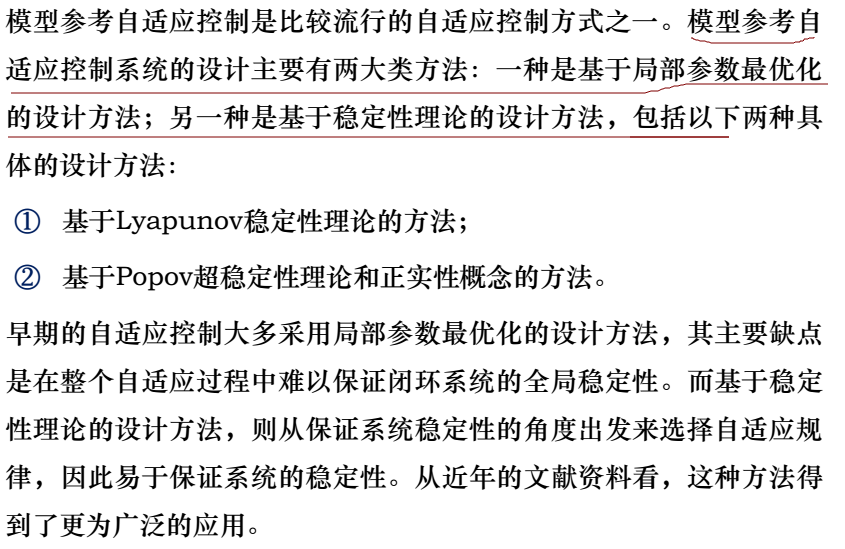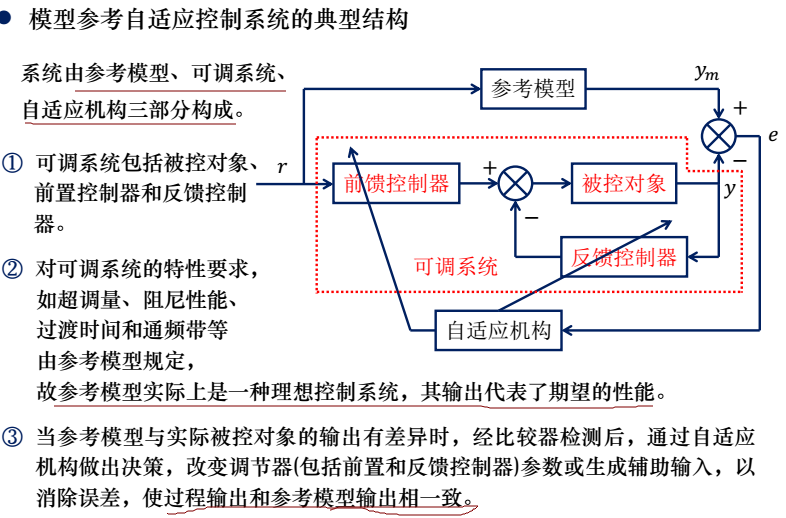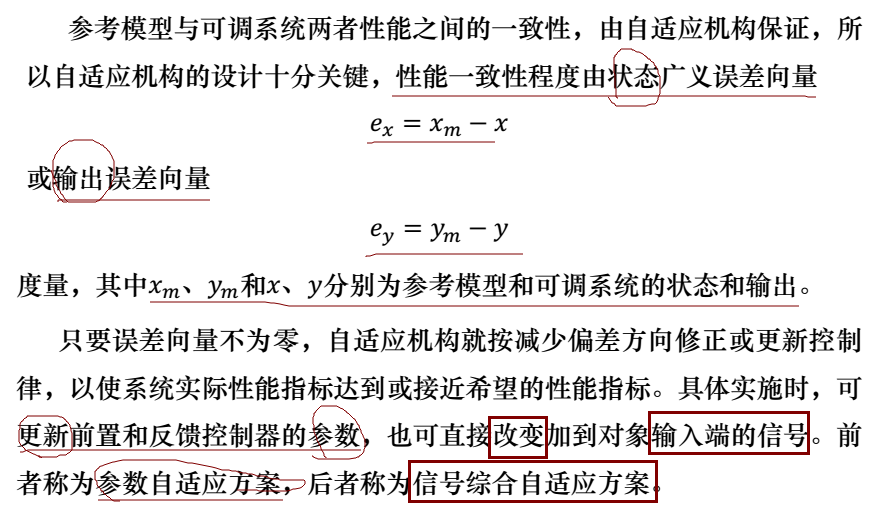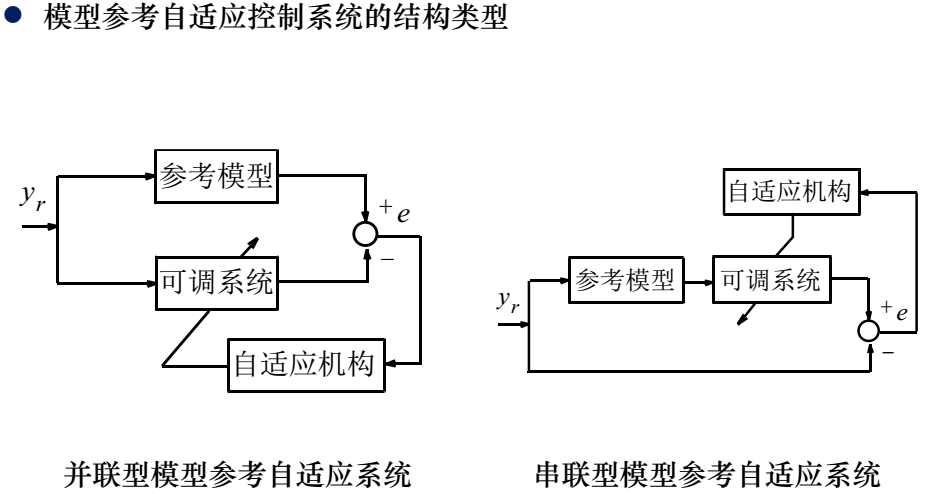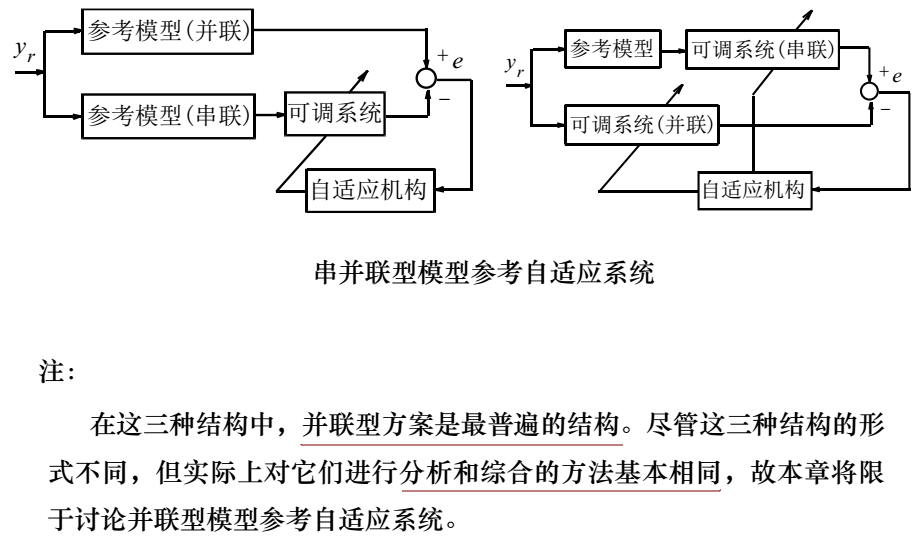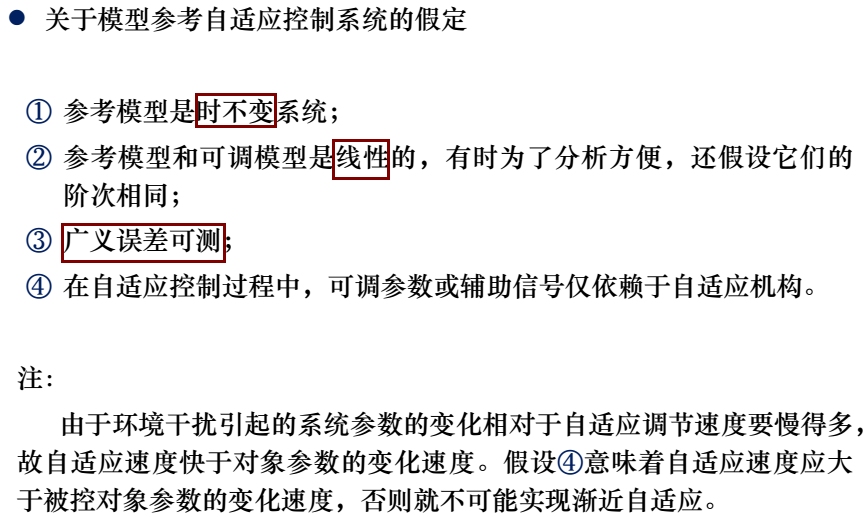基于局部参数最优化的设计方法（MIT方案）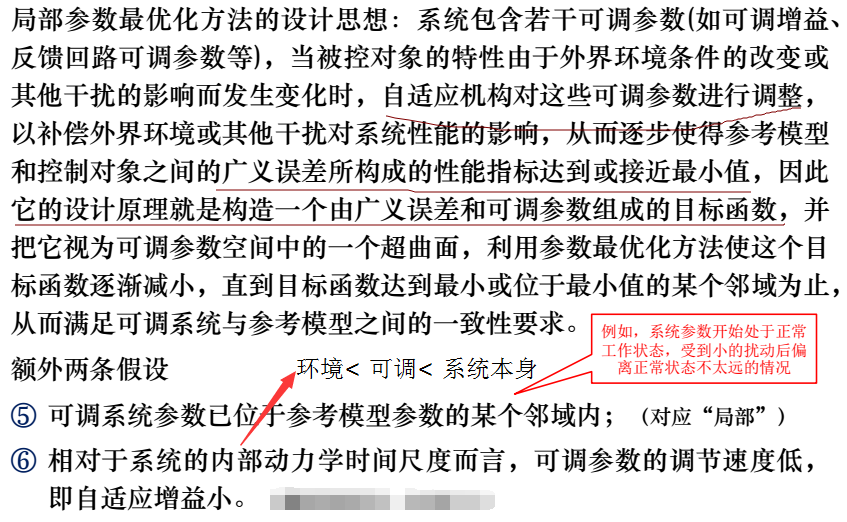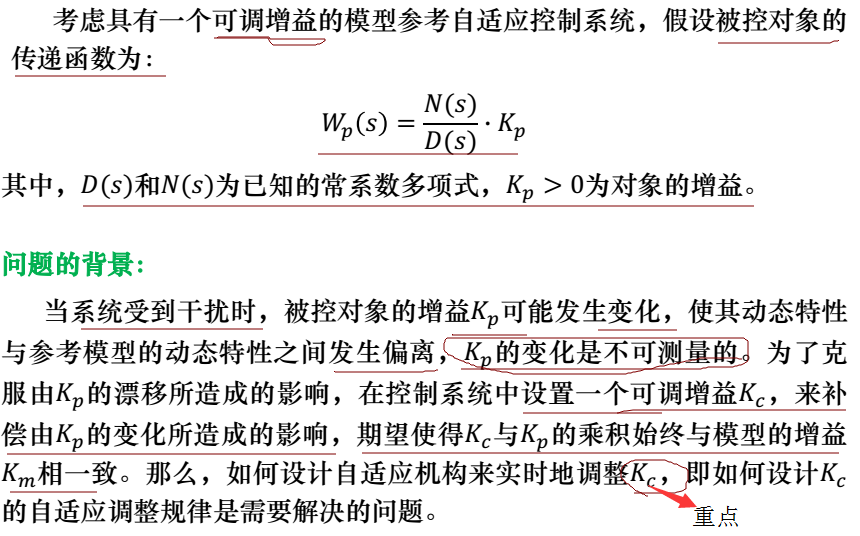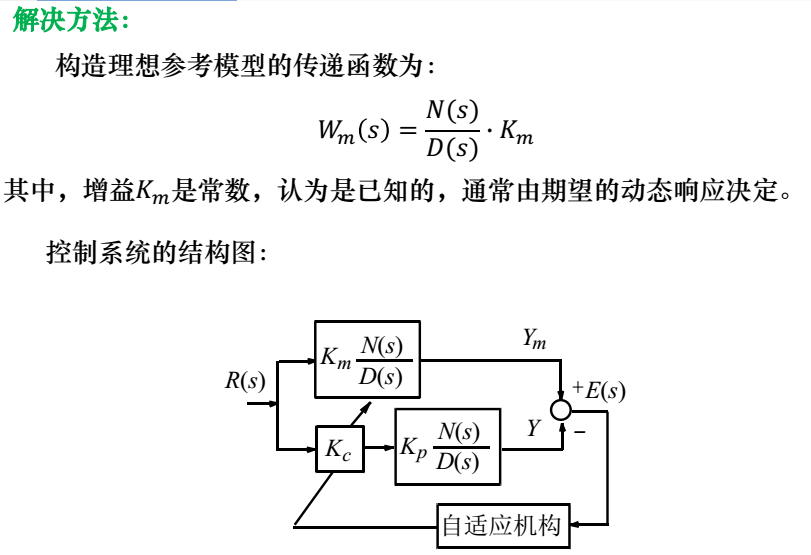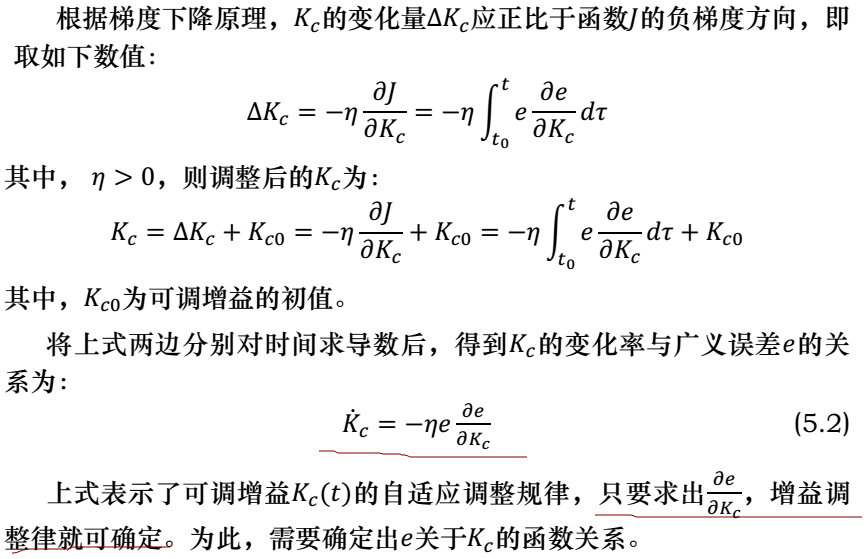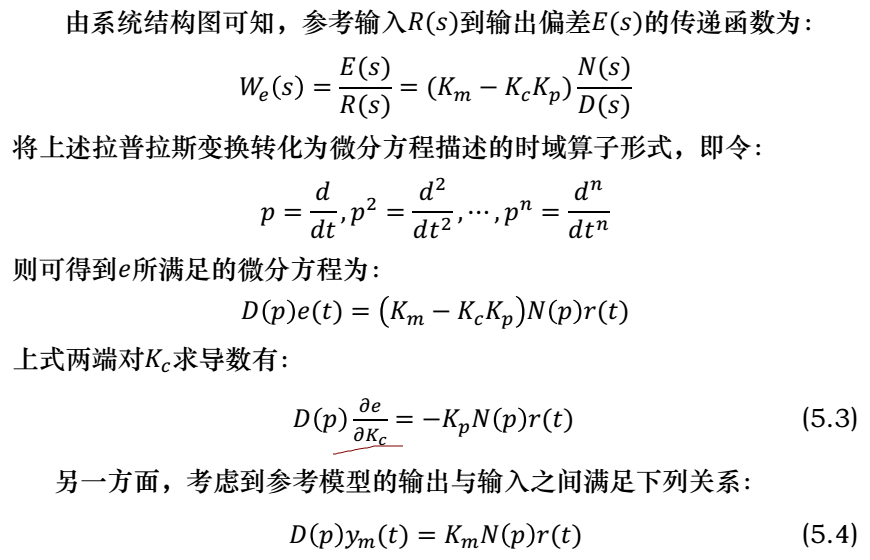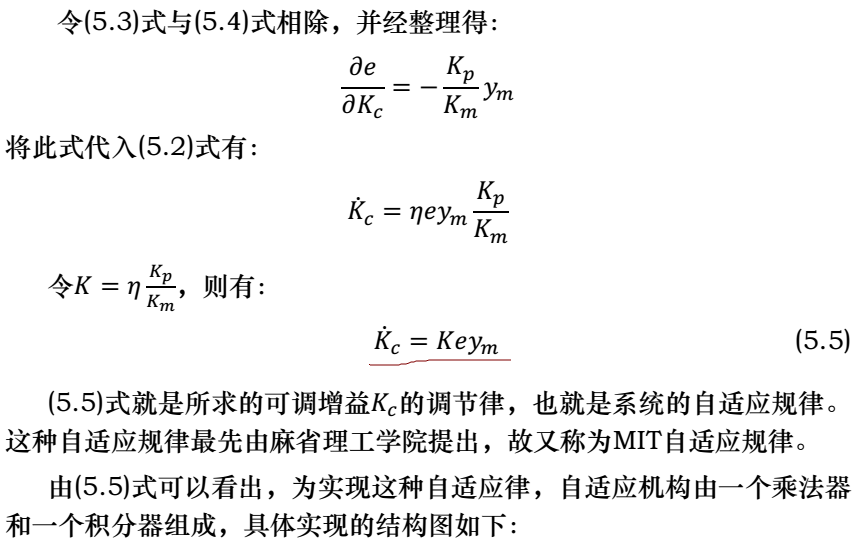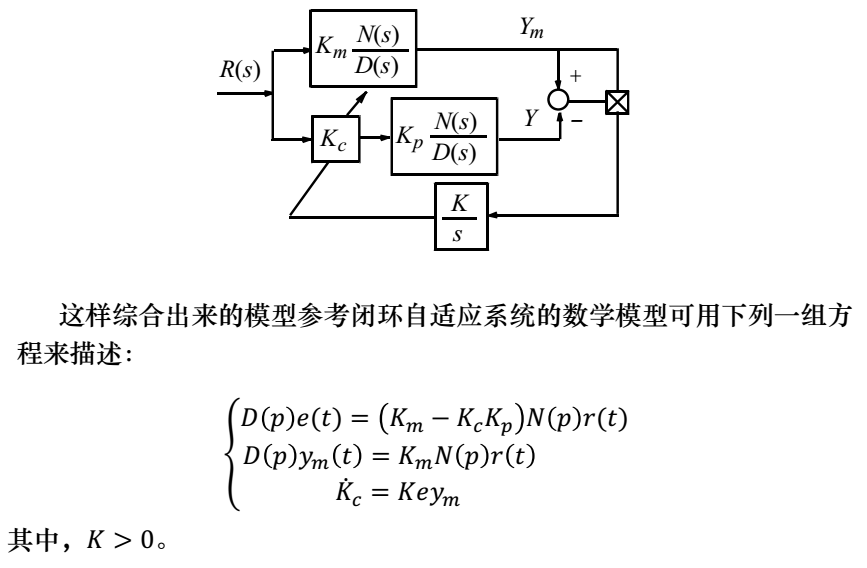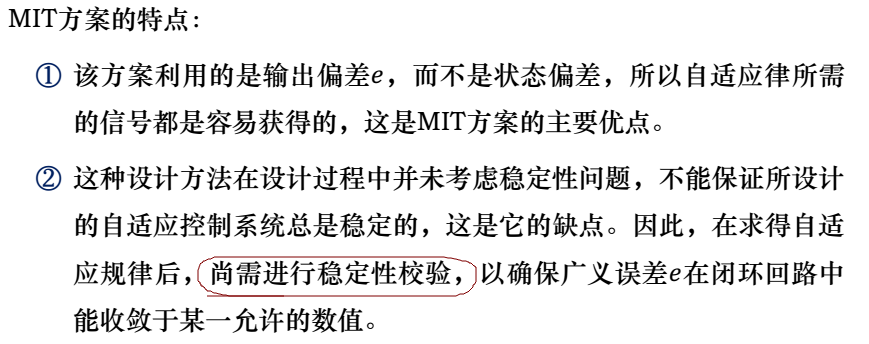举例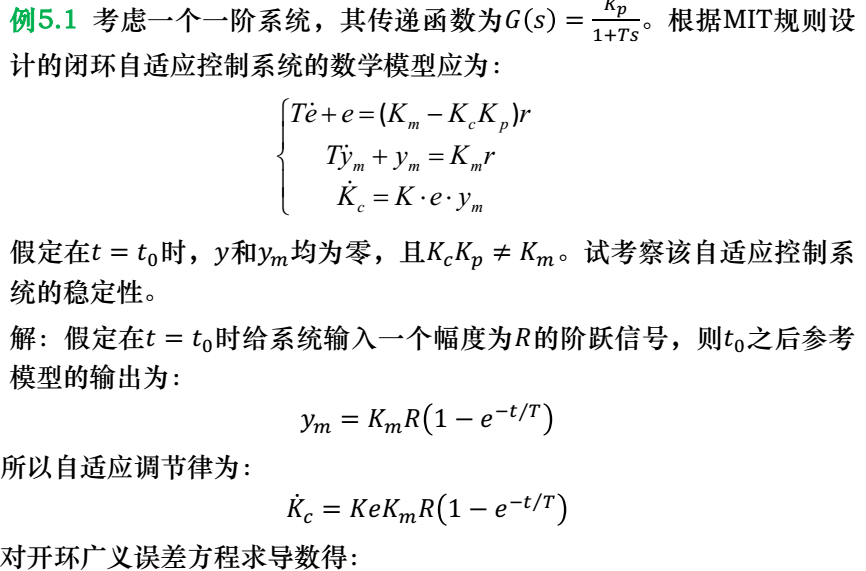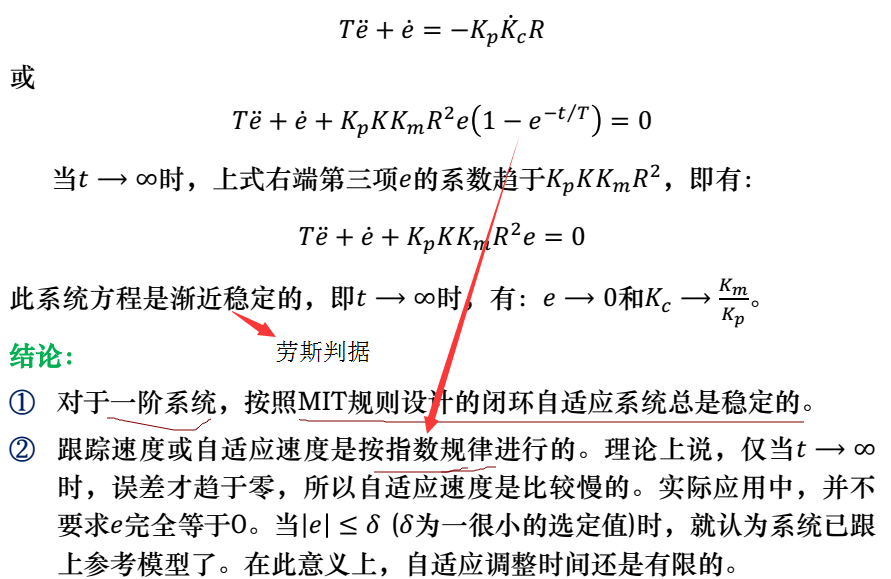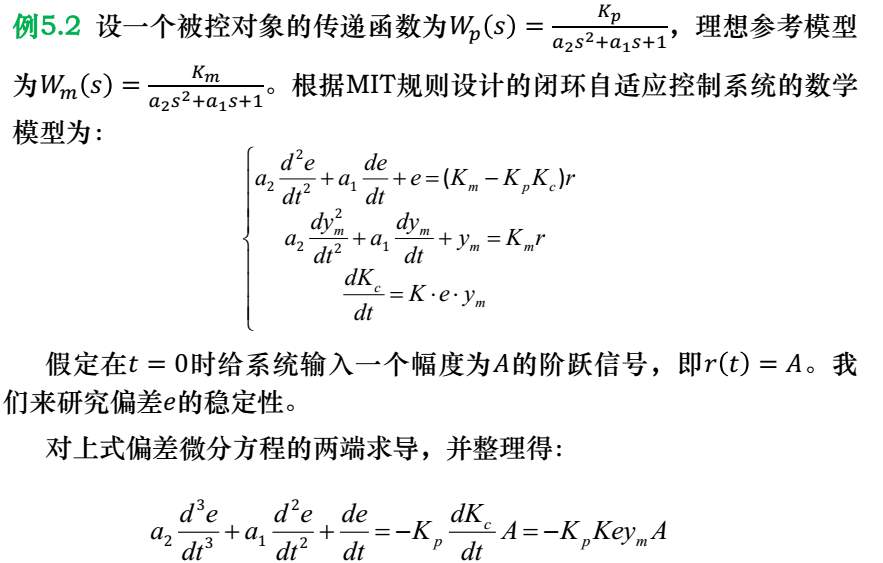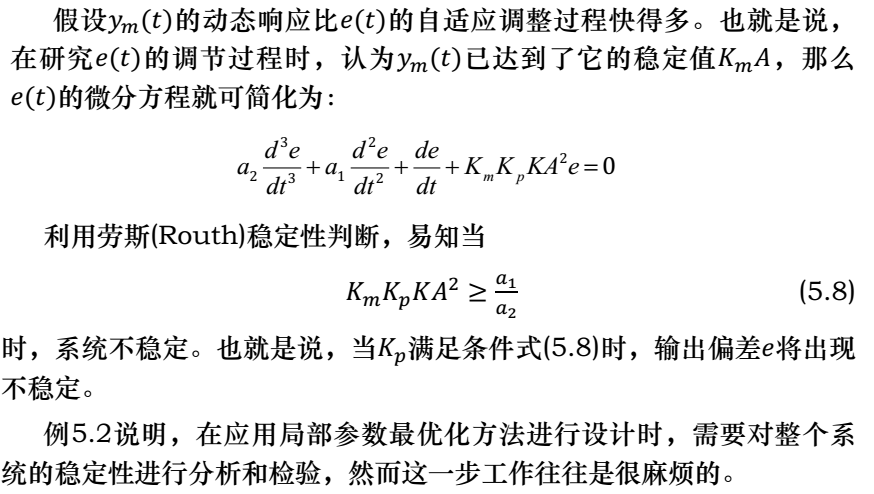n<=4时的劳斯判据：

n=1时，特征方程为：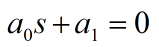，各系数为正。
n=2时，特征方程为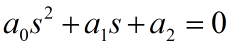，各系数为正。
n=3时，特征方程为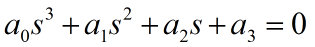，各系数为正，且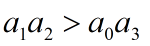。
n=4时，特征方程为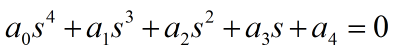，各系数为正，且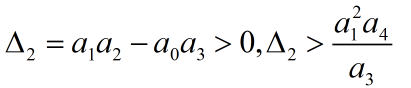。
展开全文自适应控制
• 采用三阶段启动可使电动机平稳启动，基于模型参考自适应控制（MRAC）的电动机速度检测确保了精确的换向。 最后，介绍并测试了基于数字信号控制器（DSC）的BLDCM系统的硬件和软件设计。 实验结果表明，该驱动系统...
• 不错的小论文，相信对你学自适应控制会起到比较好的作用！
• 提升模型参考自适应瞬态性能 基于上一小节的推导,我们得到的误差动力学模型为: e˙=Are−BpΛW~Tσ \dot{e}=A_re-B_p\Lambda\widetilde{W}^T\sigma e˙=Ar​e−Bp​ΛWTσ 参考模型动力学模型为: x˙r=Arxr+Brr \dot...
• Model reference adaptive controllers can guarantee both stability and performance over a wide range of slowly varying parameter changes as long as ...下面的例子是我硕士自适应控制课的三个关于Model...
• 设计了具有自动翻盖、防污除臭、温水清洗、智能存储及记忆等功能的智能坐便器控制系统；以AVR MCU为主控器，进行了控制系统的硬件和软件实现。所设计开发的控制系统已经应用于实际产品中。
• Apollo横向和纵向控制器都属于high level controller, 我们在设计控制器的过程中一直都是假设车辆的执行器是可以完美的执行我们控制算法算出来的控制命令, 然后现实是车辆执行器的延迟是非常验证的, 建议一下大家......